# Join of topological spaces

(diff) ← Older revision | Latest revision (diff) | Newer revision → (diff)
Jump to: navigation, search
This article describes a construct that involves some variant of taking a product of a topological space with the unit interval and then making some identifications, typically at the endpoints, based on some specific maps.
View more such constructs

## Definition

Given two topological spaces$X$ and$Y$, the join of$X$ and$Y$, denoted$X * Y$, is defined as follows: it is the quotient of the space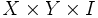$X \times Y \times I$ under the identifications: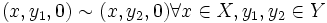$(x,y_1,0) \sim (x,y_2,0) \forall x \in X, y_1,y_2 \in Y$

and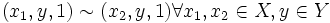$(x_1,y,1) \sim (x_2,y,1) \forall x_1,x_2 \in X, y \in Y$

Pictorially, we can think of this as the space of all line segments joining points in$X$ and$Y$, with two line segments meeting only at common endpoints.

## Particular cases

### Cone space

Further information: Cone space

The cone space of a topological space$X$ can be viewed as the join of$X$ with a one-point space.

### Suspension

Further information: suspension

The suspension of a topological space$X$ can be viewed as the join of$X$ with a two-point space.

### Simplex

The$n$-simplex can be viewed, at least topologically, as the join of$n$ one-point spaces.

## Operation properties

There is a canonical isomorphism between$X * Y$ and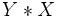$Y * X$, sending$(x,y,t)$ to (y,x,1-t)[/itex].

There is a canonical isomorphism between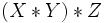$(X * Y) * Z$ and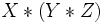$X * (Y * Z)$.